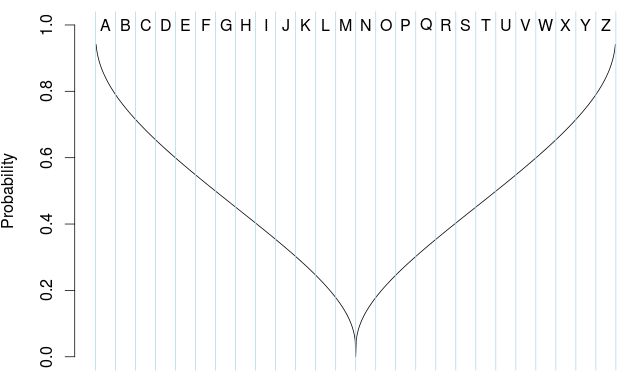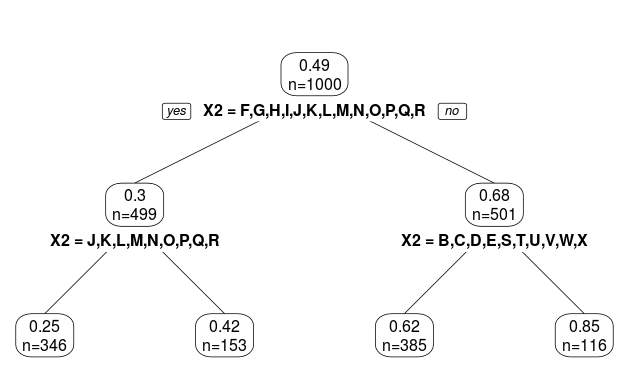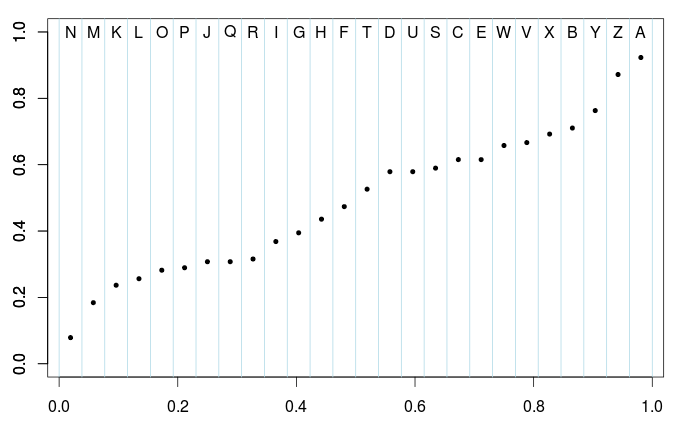Want to share your content on R-bloggers? click here if you have a blog, or here if you don't.

I think that over the past months, I have been saying non-correct things about classification with categorical covariates. Because I never took time to look at it carefuly. Consider some simulated dataset, with a logistic regression,

> n=1e3
> set.seed(1)
> X1=runif(n)
> q=quantile(X1,(0:26)/26)
> q=0
> X2=cut(X1,q,labels=LETTERS[1:26])
> p=exp(-.1+qnorm(2*(abs(.5-X1))))/(1+exp(-.1+qnorm(2*(abs(.5-X1)))))
> Y=rbinom(n,size=1,p)
> df=data.frame(X1=X1,X2=X2,p=p,Y=Y)

Here, we use some continuous covariate, except that is considered as not-observed. Instead, we have a categorical covariate with 26 categories. The (theoretical) relationship between the covariate and the probability is given below,

> vx1=seq(0,1,by=.001)
> vp=exp(-.1+qnorm(2*(abs(.5-vx1))))/(1+exp(-.1+qnorm(2*(abs(.5-vx1)))))
> plot(vx1,vp,type="l")and the empirical probability, for each modality isIf we run a classification tree, we get

> library(rpart)
> tree=rpart(Y~X2,data=df)
> library(rpart.plot)
> prp(tree, type=2, extra=1)To be more specific, the output is here

> tree
1) root 1000 249.90000 0.4900000
2) X2=F,G,H,I,J,K,L,M,N,O,P,Q,R 499 105.3 0.302
4) X2=J,K,L,M,N,O,P,Q,R 346  65.12 0.25144  *
5) X2=F,G,H,I 153  37.22876 0.4183007       *
3) X2=A,B,C,D,E,S,T,U,V,W,X,Y,Z 501 109.61 0.67
6) X2=B,C,D,E,S,T,U,V,W,X 385  90.38 0.623  *
7) X2=A,Y,Z 116  14.50862 0.8534483         *

Note that it takes less than a second to get that output. So clearly, we did not look for all combinations between modalities. For the first node, there are like  possible groups, i.e.

> 67108864

It is big… not huge, but too big to try all combinations, since that’s only the first node, and we have to do it again on the two leaves, etc. Antoine (aka @ly_antoine) told me – while we were having a coffee after lunch today – the trick to get a fast algorithm, on categories. And as usual, the idea is very clever…

First, we need a function to compute Gini index

> gini=function(y,classe){
+    T=table(y,classe)
+    nx=apply(T,2,sum)
+    n=sum(T)
+    pxy=T/matrix(rep(nx,each=2),nrow=2)
+    omega=matrix(rep(nx,each=2),nrow=2)/n
+    g=-sum(omega*pxy*(1-pxy))
+    return(g)}

For the first node, the idea is very simple:

• Compute empirical averages
> cond_prob=aggregate(df$Y,by=list(df$X2),mean)
• Then sort those values, ,
• Based on that ordering, consider
> Group_Letters=cond_prob[order(cond_prob$x),2]• Then consider (only) possible partitions, against > v_gini=rep(NA,26) > for(v in 1:26){ + CLASSE=df$X2 %in% Group_Letters[1:v]
+   v_gini[v]=gini(y=df$Y,classe=CLASSE) + } If we plot them, we get > plot(1:26,v_gini,type="b)As for continuous variables, we seek for the maximum value, and then, we have our two groups,> sort(Group_Letters[1:which.max(v_gini)])  F G H I J K L M N O P Q R That’s exactly what we got with the tree function in R, 1) root 1000 249.90000 0.4900000 2) X2=F,G,H,I,J,K,L,M,N,O,P,Q,R 499 105.30 0.30 Now, consider the leaf on the left (for instance) > sub_df=df[df$X2 %in% sort(Group_Letters[1:which.max(v_gini)]),]

Then use the same algorithm as before: sort the conditional means,

> cond_prob=aggregate(sub_df$Y,by= + list(sub_df$X2),mean)
> s_Group_Letters=cond_prob[order(cond_prob$x),2] Then compute Gini indices based on groups obtained from that ordering, > v_gini=rep(NA,length(sub_Group_Letters)) > for(v in 1:length(sub_Group_Letters)){ + CLASSE=sub_df$X2 %in% s_Group_Letters[1:v]
+   v_gini[v]=gini(y=sub_df\$Y,classe=CLASSE)
+ }

If we plot it, we get our two groups,

> plot(1:length(s_Group_Letters),v_gini,type="b")And the first group is here

> sort(sub_Group_Letters[1:which.max(v_gini)])
 J K L M N O P Q R

Again, that’s exactly what we got with the R function

1) root 1000 249.90000 0.4900000
2) X2=F,G,H,I,J,K,L,M,N,O,P,Q,R 499 105.30 0.30
4) X2=J,K,L,M,N,O,P,Q,R 346  65.12 0.25144  *

Clever, isn’t?